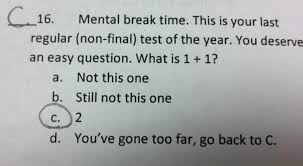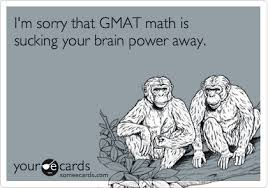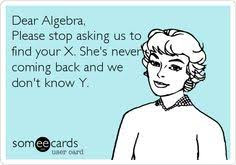## Free and New GMAT Practice Test Sample Questions

### GMAT Practice Test Sample Questions

This is my NEW blog on GMAT free practice questions. In the first blog we saw how to prepare for GMAT. In this blog you will some more practice questions. You can find all answer in the middle of blog.Q 1

Which of the following is not a whole number followed by its square?

A.1, 1

B.6, 36

C.8, 64

D.10, 100

E.11, 144

Q 2

Describe the following sequence in mathematical terms. 144, 72, 36, 18, 9

A.Descending arithmetic sequence

B.Ascending arithmetic sequence

C.Descending geometric sequence

D.Ascending geometric sequence

E.Miscellaneous sequence

Q 3

A nurse has to record her temperatures in Celsius but her thermometer reads Fahrenheit. A patient's temperature is 100.7° F. What is the temperature in °C?

A.32° C

B.36.5° C

C.38.2° C

D.213.3° C

E.223.7° C

Q 4

Art realized that he had 2 more quarters than he had originally thought in his pocket. If all of the change in his pocket is quarters and it totals to \$8.75, how many quarters did he originally think were in his pocket?

A.27

B.29

C.31

D.33

E.35

Q 5

There are 12 more apples than oranges in a basket of 36 apples and oranges. How many apples are in the basket?

A.12

B.15

C.24

D.28

E.36Q 6

Which of the following correctly identifies 4 consecutive odd integers where the sum of the middle two integers is equal to 24?

A.5, 7, 9, 11

B.7, 9, 11, 13

C.9, 11, 13, 15

D.11, 13, 15, 17

E.13, 15, 17, 19

Q 7

What is the next number in the sequence? 6, 12, 24, 48, ___

A.72

B.96

C.108

D.112

E.124

Q 8

Which of the following numbers could be described in the following way: an integer that is a natural, rational and whole number?

A.0

B.1

C.2.33

D.-3

E. None of the above

Q 9

What is the next number in the following pattern? 1, 1/2, 1/4, 1/8, ___

A.1/10

B.1/12

C.1/14

D.1/15

E.1/16Q 10

Of the following units, which would be most likely to measure the amount of sugar needed in a recipe for 2 dozen cookies

A.degrees Celsius

B.milliliters

C.quarts

D.kilograms

E.cups

### Answers & Explanations GMAT Practice Test Sample Questions 1-10

6=1. E: 112=121, not 144.

22. C: The descending sequence is geometric, with a common ratio of 0.5.

3. C: The conversion formula is: C=(F-32).5/9, where C represents degrees Celsius and F represents degrees Fahrenheit. Substituting 100.7 for F gives: C=(100.7-32).5/9, which simplifies to C=68.7.5/9. Thus, the temperature, in Celsius, is approximately 38.2°.

4. D: The problem may be modeled by the equation, 0.25x = 8.75. Solving for x gives x = 35. Since he thought he had 2 fewer quarters, he originally thought he had 33 quarters in his pocket.

5. C: The problem may be modeled by the following system of equations:(a=o+12@a+o=36). Substituting the expression for a, into the second equation, gives: o + 12 + o = 36. Solving for o gives o = 12. Thus, there are 12 oranges. Since there are 36 apples and oranges in all, there must be 24 apples.

6. C: The sequence, 9, 11, 13, 15, shows all odd integers, which are consecutive. The sum of 11 and 13 is indeed 24.

7. B: The sequence is a geometric sequence, with a common ratio of 2. Two times 48 is 96, thus the next number in the sequence is 96.

8. B: The number, 1, is rational, whole, and natural. A rational number is a number that terminates or repeats. A whole number is represented by the sequence, 0, 1, 2, 3, 4, …, while a natural number is a subset of the whole numbers, and is represented by the sequence, 1, 2, 3, 4,...

9. E: The sequence is a geometric sequence, with a common ratio of 1/2. Multiplication of 1/8 by 1/2 gives 1/16, which is the next number in the sequence.

10. E: The amount of sugar, needed in a cookie recipe, is best measured by the unit of cups, which is an appropriate measure of capacity.